Updating search results...# Grade 9 DLC Blended Math

This collection features resources developed by The Distance Learning Centre (DLC) for blended math in Grade 9.

44 affiliated resources

# Search Resources

View
Selected filters: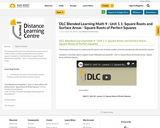Conditional Remix & Share Permitted
CC BY-NC-SA
Rating
0.0 stars

The purpose of this lesson is to determine the square roots of whole numbers, fractions and decimals that are perfect squares.

Included is a YouTube video to support Grade 9 Blended Learning Math - Unit 1.1: Square Roots and Surface Areas.

Subject:
Math
Material Type:
Activity/Lab
Homework/Assignment
Lesson
Provider:
Sun West Distance Learning Centre (DLC)
12/05/2023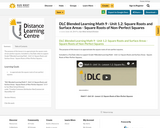Conditional Remix & Share Permitted
CC BY-NC-SA
Rating
0.0 stars

The purpose of this lesson is to approximate the square roots of non-perfect squares.

Included is a YouTube video to support Grade 9 Blended Learning Math - Unit 1.2: Square Roots and Surface Areas - Square Roots of Non-Perfect Squares.

Subject:
Math
Material Type:
Activity/Lab
Homework/Assignment
Lesson
Provider:
Sun West Distance Learning Centre (DLC)
12/05/2023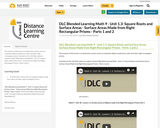Conditional Remix & Share Permitted
CC BY-NC-SA
Rating
0.0 stars

The purpose of this lesson is to determine surface areas of composite objects made from cubes and other right rectangular prisms.

Included are the YouTube videos to support Grade 9 Blended Learning Math - Unit 1.3: Square Roots and Surface Areas - Surface Areas Made from Right Rectangular Prisms - Parts 1 and 2.

Subject:
Math
Material Type:
Activity/Lab
Homework/Assignment
Lesson
Provider:
Sun West Distance Learning Centre (DLC)
12/05/2023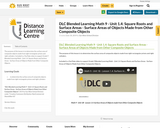Conditional Remix & Share Permitted
CC BY-NC-SA
Rating
0.0 stars

The purpose of this lesson is to determine the surface area of composite objects made from right rectangular prisms and right cylinders.

Included is a YouTube video to support Grade 9 Blended Learning Math - Unit 1.4: Square Roots and Surface Areas - Surface Areas of Objects Made from Other Composite Objects.

Subject:
Math
Material Type:
Activity/Lab
Homework/Assignment
Lesson
Provider:
Sun West Distance Learning Centre (DLC)
12/05/2023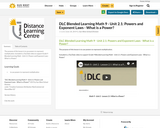Conditional Remix & Share Permitted
CC BY-NC-SA
Rating
0.0 stars

The purpose of this lesson is to use powers to represent multiplication.

Included is a YouTube video to support Grade 9 Blended Learning Math - Unit 2.1: Powers and Exponent Laws - What is a Power?

Subject:
Math
Material Type:
Activity/Lab
Homework/Assignment
Lesson
Provider:
Sun West Distance Learning Centre (DLC)
12/05/2023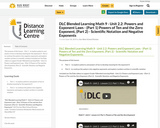Conditional Remix & Share Permitted
CC BY-NC-SA
Rating
0.0 stars

The purpose of this lesson:
- Part 1 - to explore patterns and powers of ten to develop meaning for the exponent 0
- Part 2 - to continue the pattern into negative exponents and explore numbers written in scientific notation
Included are YouTube videos to support Grade 9 Blended Learning Math - Unit 2.2: Powers and Exponent Laws - (Part 1) Powers of Ten and the Zero Exponent, (Part 2) - Scientific Notation and Negative Exponents.

Subject:
Math
Material Type:
Activity/Lab
Homework/Assignment
Lesson
Provider:
Sun West Distance Learning Centre (DLC)
12/05/2023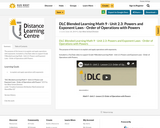Conditional Remix & Share Permitted
CC BY-NC-SA
Rating
0.0 stars

The purpose of this lesson is to explain and apply operations with exponents.

Included is a YouTube video to support Grade 9 Blended Learning Math - Unit 2.3: Powers and Exponent Laws - Order of Operations with Powers.

Subject:
Math
Material Type:
Activity/Lab
Homework/Assignment
Lesson
Provider:
Sun West Distance Learning Centre (DLC)
12/05/2023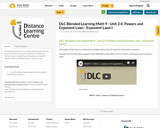Conditional Remix & Share Permitted
CC BY-NC-SA
Rating
0.0 stars

The purpose of this lesson is to understand and apply exponent laws for products and quotients of powers.

Included is the YouTube video to support Grade 9 Blended Learning Math - Unit 2.4: Powers and Exponent Laws - Exponent Laws I.

Subject:
Math
Material Type:
Activity/Lab
Homework/Assignment
Lesson
Provider:
Sun West Distance Learning Centre (DLC)
12/05/2023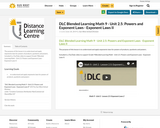Conditional Remix & Share Permitted
CC BY-NC-SA
Rating
0.0 stars

The purpose of this lesson is to understand and apply exponents laws for powers of products, quotients and powers.

Included is a YouTube video to support Grade 9 Blended Learning Math - Unit 2.5: Powers and Exponent Laws - Exponent Laws II.

Subject:
Math
Material Type:
Activity/Lab
Homework/Assignment
Lesson
Provider:
Sun West Distance Learning Centre (DLC)
12/05/2023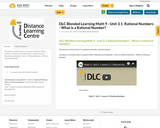Conditional Remix & Share Permitted
CC BY-NC-SA
Rating
0.0 stars

The purpose of this lesson is to compare and order rational numbers.

Included is a YouTube video to support Grade 9 Blended Learning Math - Unit 3.1: Rational Numbers - What is a Rational Number?

Subject:
Math
Material Type:
Activity/Lab
Homework/Assignment
Lesson
Provider:
Sun West Distance Learning Centre (DLC)
12/05/2023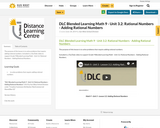Conditional Remix & Share Permitted
CC BY-NC-SA
Rating
0.0 stars

The purpose of this lesson is to solve problems that require adding rational numbers.

Included is a YouTube video to support Grade 9 Blended Learning Math - Unit 3.2: Rational Numbers - Adding Rational Numbers.

Subject:
Math
Material Type:
Activity/Lab
Homework/Assignment
Lesson
Provider:
Sun West Distance Learning Centre (DLC)
12/05/2023Conditional Remix & Share Permitted
CC BY-NC-SA
Rating
0.0 stars

The purpose of this lesson is to solve problems that involve subtracting rational numbers.

Included is a YouTube video to support Grade 9 Blended Learning Math - Unit 3.3: Rational Numbers - Subtracting Rational Numbers.

Subject:
Math
Material Type:
Activity/Lab
Homework/Assignment
Lesson
Provider:
Sun West Distance Learning Centre (DLC)
12/05/2023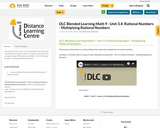Conditional Remix & Share Permitted
CC BY-NC-SA
Rating
0.0 stars

The purpose of this lesson is to solve problems that require the multiplication of rational numbers.

Included is a YouTube video to support Grade 9 Blended Learning Math - Unit 3.4: Rational Numbers - Multiplying Rational Numbers.

Subject:
Math
Material Type:
Activity/Lab
Homework/Assignment
Lesson
Provider:
Sun West Distance Learning Centre (DLC)
12/05/2023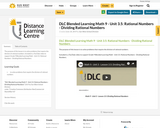Conditional Remix & Share Permitted
CC BY-NC-SA
Rating
0.0 stars

The purpose of this lesson is to solve problems that require the division of rational numbers.

Included is a YouTube video to support Grade 9 Blended Learning Math - Unit 3.5: Rational Numbers - Dividing Rational Numbers.

Subject:
Math
Material Type:
Activity/Lab
Homework/Assignment
Lesson
Provider:
Sun West Distance Learning Centre (DLC)
12/05/2023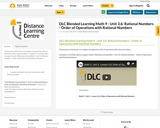Conditional Remix & Share Permitted
CC BY-NC-SA
Rating
0.0 stars

The purpose of this lesson is to explain and apply the order of operations with rational numbers.

Included is a YouTube video to support Grade 9 Blended Learning Math - Unit 3.6: Rational Numbers - Order of Operations with Rational Numbers.

Subject:
Math
Material Type:
Activity/Lab
Homework/Assignment
Lesson
Provider:
Sun West Distance Learning Centre (DLC)
12/05/2023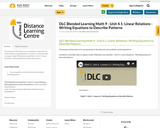Conditional Remix & Share Permitted
CC BY-NC-SA
Rating
0.0 stars

The purpose of this lesson is to use equations to describe and solve problems involving patterns.

Included is a YouTube video to support Grade 9 Blended Learning Math - Unit 4.1: Linear Equations - Writing Equations to Describe Patterns.

Subject:
Math
Material Type:
Activity/Lab
Homework/Assignment
Lesson
Provider:
Sun West Distance Learning Centre (DLC)
12/05/2023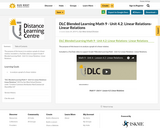Conditional Remix & Share Permitted
CC BY-NC-SA
Rating
0.0 stars

The purpose of this lesson is to analyze a graph of a linear relation.

Included is a YouTube video to support Grade 9 Blended Learning Math - Unit 4.2: Linear Relations- Linear Relations.

Subject:
Math
Material Type:
Activity/Lab
Homework/Assignment
Lesson
Provider:
Sun West Distance Learning Centre (DLC)
12/05/2023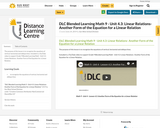Conditional Remix & Share Permitted
CC BY-NC-SA
Rating
0.0 stars

The purpose of this lesson is to recognize the equations of vertical, horizontal and oblique lines.

Included is a YouTube video to support Grade 9 Blended Learning Math - Unit 4.3: Linear Relations- Another Form of the Equation for a Linear Relation.

Subject:
Math
Material Type:
Activity/Lab
Homework/Assignment
Lesson
Provider:
Sun West Distance Learning Centre (DLC)
12/05/2023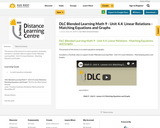Conditional Remix & Share Permitted
CC BY-NC-SA
Rating
0.0 stars

The purpose of this lesson is to match equations and graphs.

Included is a YouTube video to support Grade 9 Blended Learning Math - Unit 4.4: Linear Relations - Matching Equations and Graphs.

Subject:
Math
Material Type:
Activity/Lab
Homework/Assignment
Lesson
Provider:
Sun West Distance Learning Centre (DLC)
12/05/2023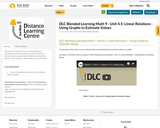Conditional Remix & Share Permitted
CC BY-NC-SA
Rating
0.0 stars

The purpose of this lesson is to use interpolation and extrapolation to estimate values on a graph.

Included is a YouTube video to support Grade 9 Blended Learning Math - Unit 4.5: Linear Relations - Using Graphs to Estimate Values.

Subject:
Math
Material Type:
Activity/Lab
Homework/Assignment
Lesson
Provider:
Sun West Distance Learning Centre (DLC)